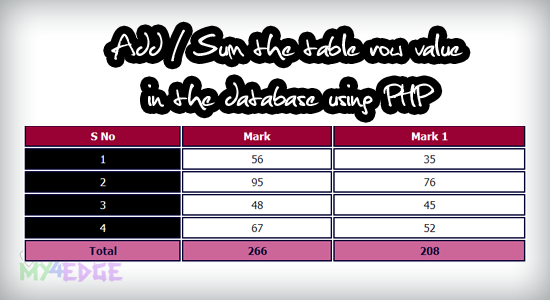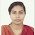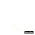Add / SUM the table row values in database using PHP | 2my4edge

## 19 December 2013

### Add / SUM the table row values in database using PHP

Here i'm going to tell about, how to make addition or sum the table row values in the mysql database using PHP, this concept is very simple one, let see how to make this addition in mysql database using PHP.you know this, like the above sample image is the demo of the add or sum the database data values in the database using PHP, you can see the out put value in the last row the table. ok let see the code.

DATABASE
```<?php
\$conn=mysql_connect('localhost','root','');
\$db=mysql_select_db('2my4edge',\$conn);
?>
```

CODE
```<table>
<tr>
</tr>

<?php
include('db.php');
while(\$row=mysql_fetch_array(\$table))
{
\$number=\$row['id'];
\$smark=\$row['mark'];
\$smark1=\$row['mark1'];
?>
<tr>
<td class="sno"><?php echo \$number ?></td>
<td class="mark"><?php echo \$smark ?></td>
<td class="mark"><?php echo \$smark1 ?></td>
</tr>
<?php } ?>

<?php
{
\$mark=\$row1['SUM(mark)'];
\$mark1=\$row1['SUM(mark1)'];
?>
<tr>
<td class="foot">Total</td>
<td class="foot"><?php echo \$mark ?></td>
<td class="foot"><?php echo \$mark1 ?></td>
</tr>
<?php } ?>
</table>
```

i hope you can get clear idea from the above code. SUM(column-name) is very important. i hope this is very useful to everyone. Thank you.

RELATED POST :

1.Arun kumar is back on fire :)

2.This is great, Thank you

--The word impossible says “I'm Possible”--

3.thats great solution. Thanks a lot. God Bless U.

4.Thanks for the coding ya.........

5.Good one........................

6.This was very helpful ........ Thank you

7.I have a similar problem to solve, only that the summation has to be along the row. Say the Total is a column head and we needed to have the total of Row 1 for Mark 1, Mark 2 and Mark3 as below

STUDENT Mark 1 Mark 2 Mark 3 Total
John 45 50 49
Milton 44 50 47
Paul 48 45 49

Any suggestions?

8.9.database bhi de diya karo

10.nice one.clear concept.thanks

11.They have error show in this coding

12.Wah Bhai, Gazab ka tutorial He, yaar.......